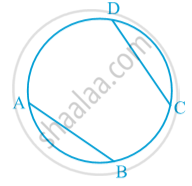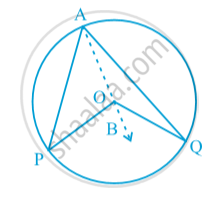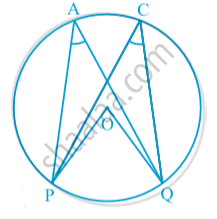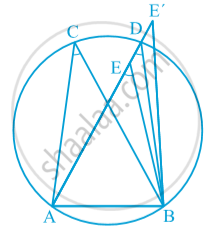# Angle Subtended by an Arc of a Circle

#### notes

If two chords of a circle are equal, then their corresponding arcs are congruent and conversely, if two arcs are congruent, then their corresponding chords are equal. In following fig.Conversely, If two arcs are equal or congruent then their corresponding chords are equal.
AB ≅ CD
=> AB = CD

#### theorem

Theorem: The angle subtended by an arc at the centre is double the angle subtended by it at any point on the remaining part of the circle.If in a circle with centre O , arc PQ of a circle subtends ∠POQ at centre and arc PQ subtends ∠PAQ on the remaining part of the circle than  ∠POQ = 2 PAQ.

Theorem: Angles in the same segment of a circle are equal.
Suppose we join points P and Q and form a chord PQ in the above figures. Then ∠ PAQ is also called the angle formed in the segment PAQP.∠ POQ = 2 ∠ PCQ = 2 ∠ PAQ
Therefore, ∠ PCQ = ∠ PAQ.
Here ∠PAQ is an angle in the segment, which is a semicircle. Also, ∠ PAQ = 1/2
∠POQ = 1/2 xx = 180° =90°

Theorem:   If a line segment joining two points subtends equal angles at two other points lying on the same side of the line containing the line segment, the four points lie on a circle (i.e. they are concyclic).
In following fig. AB is a line segment, which subtends equal angles at two points C and D. That is ∠ ACB = ∠ ADBTo show that the points A, B, C and D lie on a circle. let us draw a circle through the points A, C and B. Suppose it does not pass through the point D. Then it will intersect AD (or extended AD) at a point, say E .
If points A, C, E and B lie on a circle,
∠ ACB = ∠ AEB
But it is given that ∠ ACB = ∠ ADB.
Therefore, ∠ AEB = ∠ ADB.
This is not possible unless E coincides with D.
Similarly, E′ should also coincide with D.

If you would like to contribute notes or other learning material, please submit them using the button below.

### Shaalaa.com

Theorem : The angle subtended by an arc at the centre is double the angle subtended by it at any point on the remaining part of the circle. [00:13:32]
S